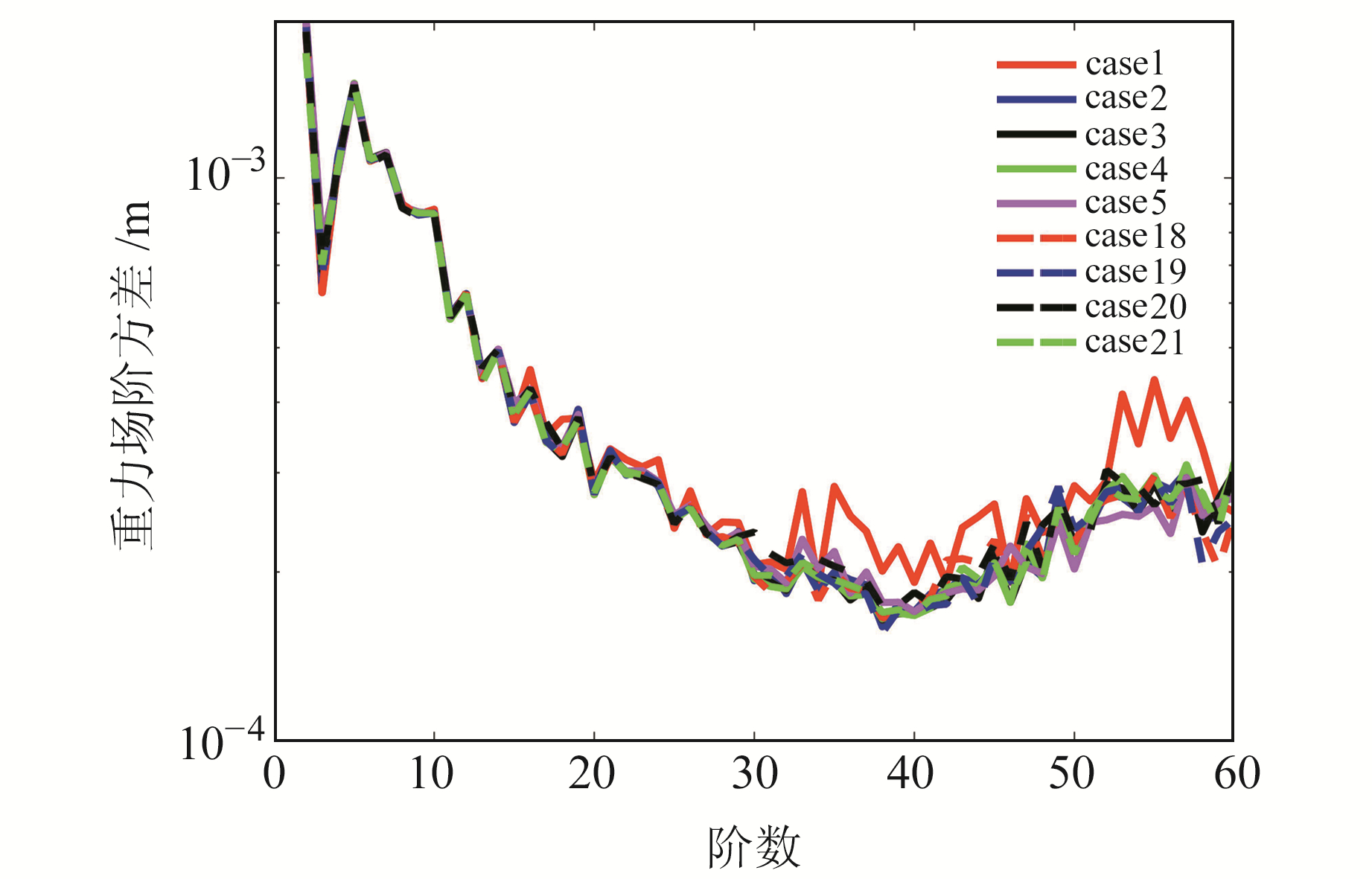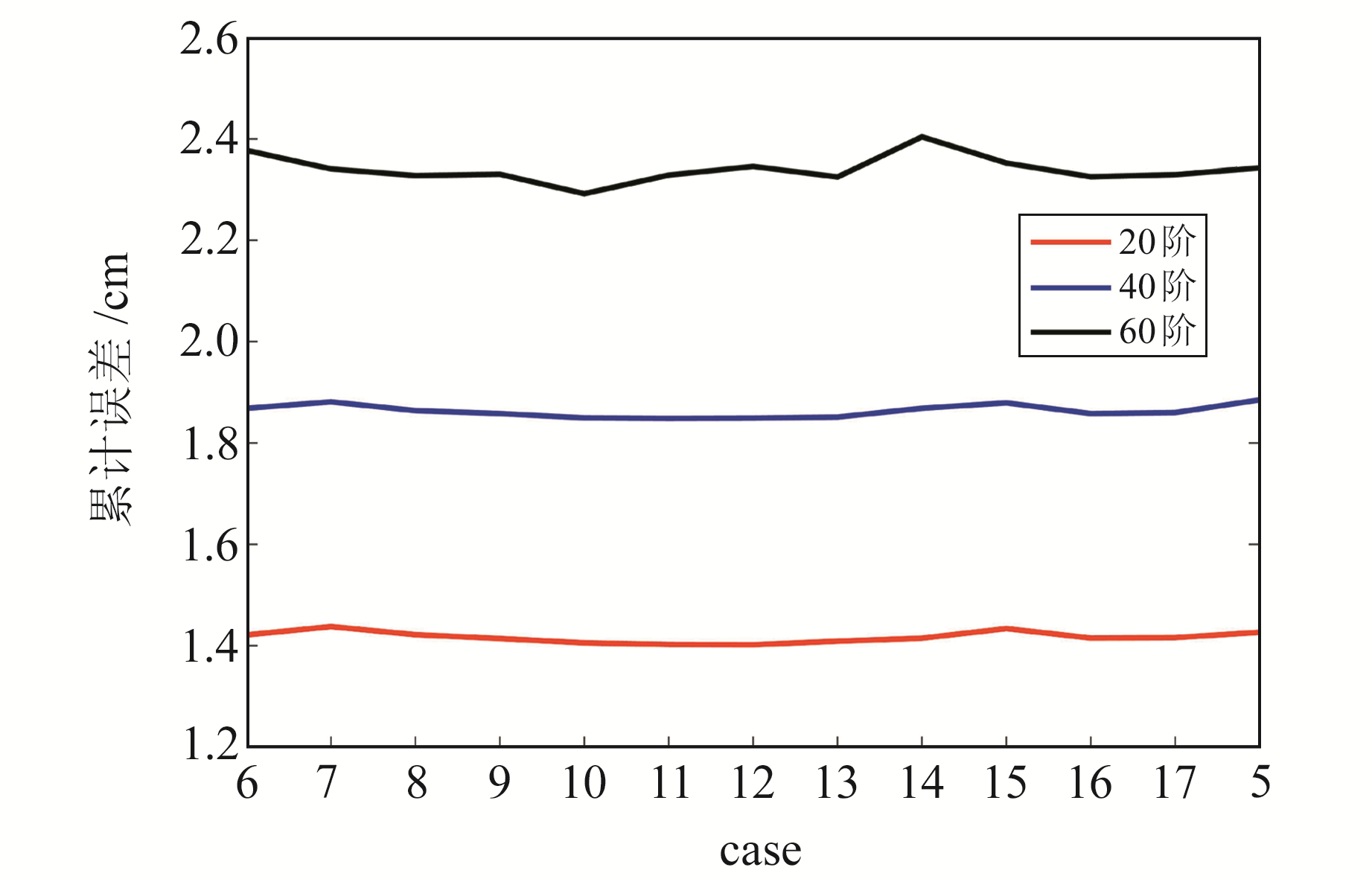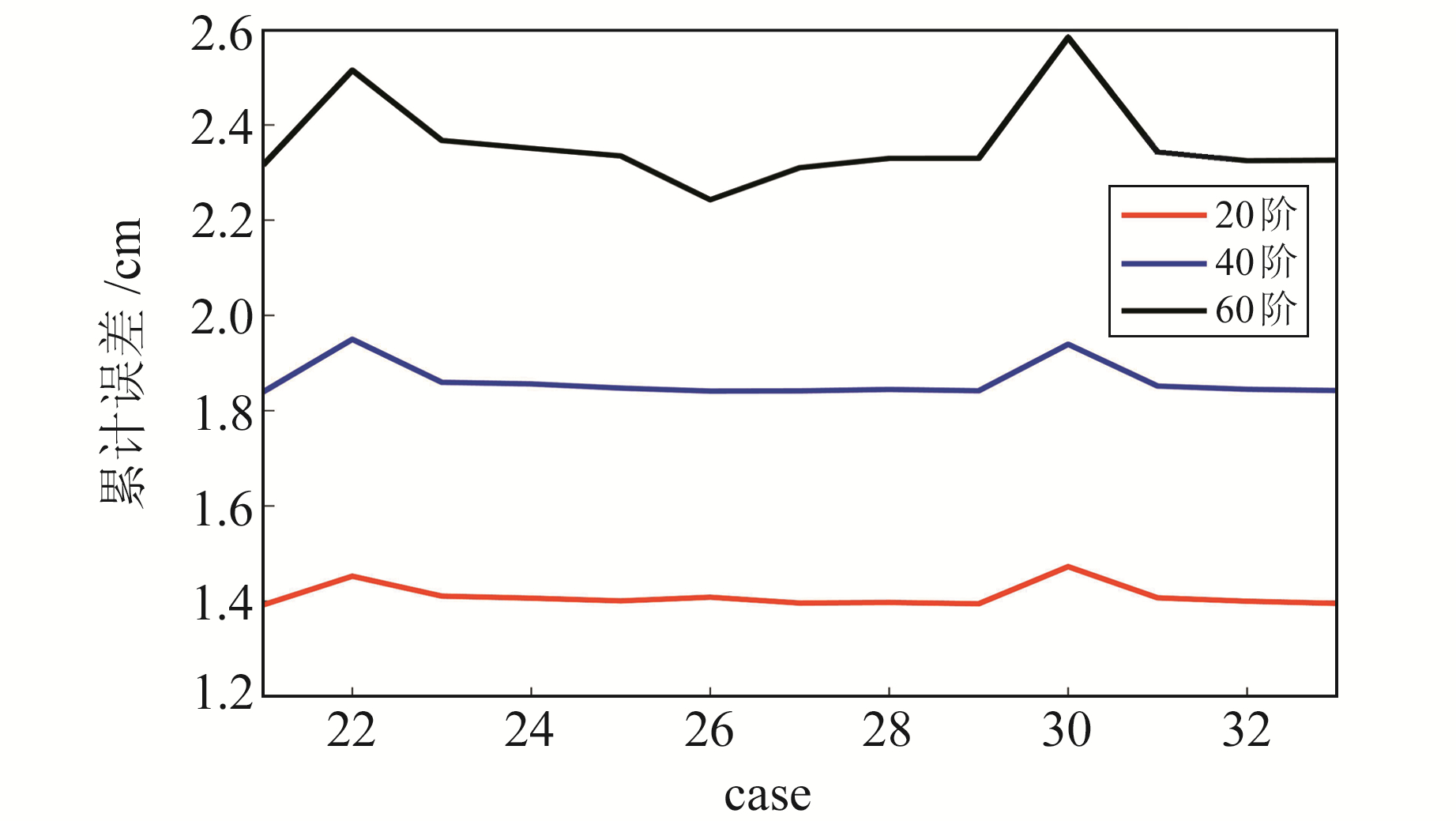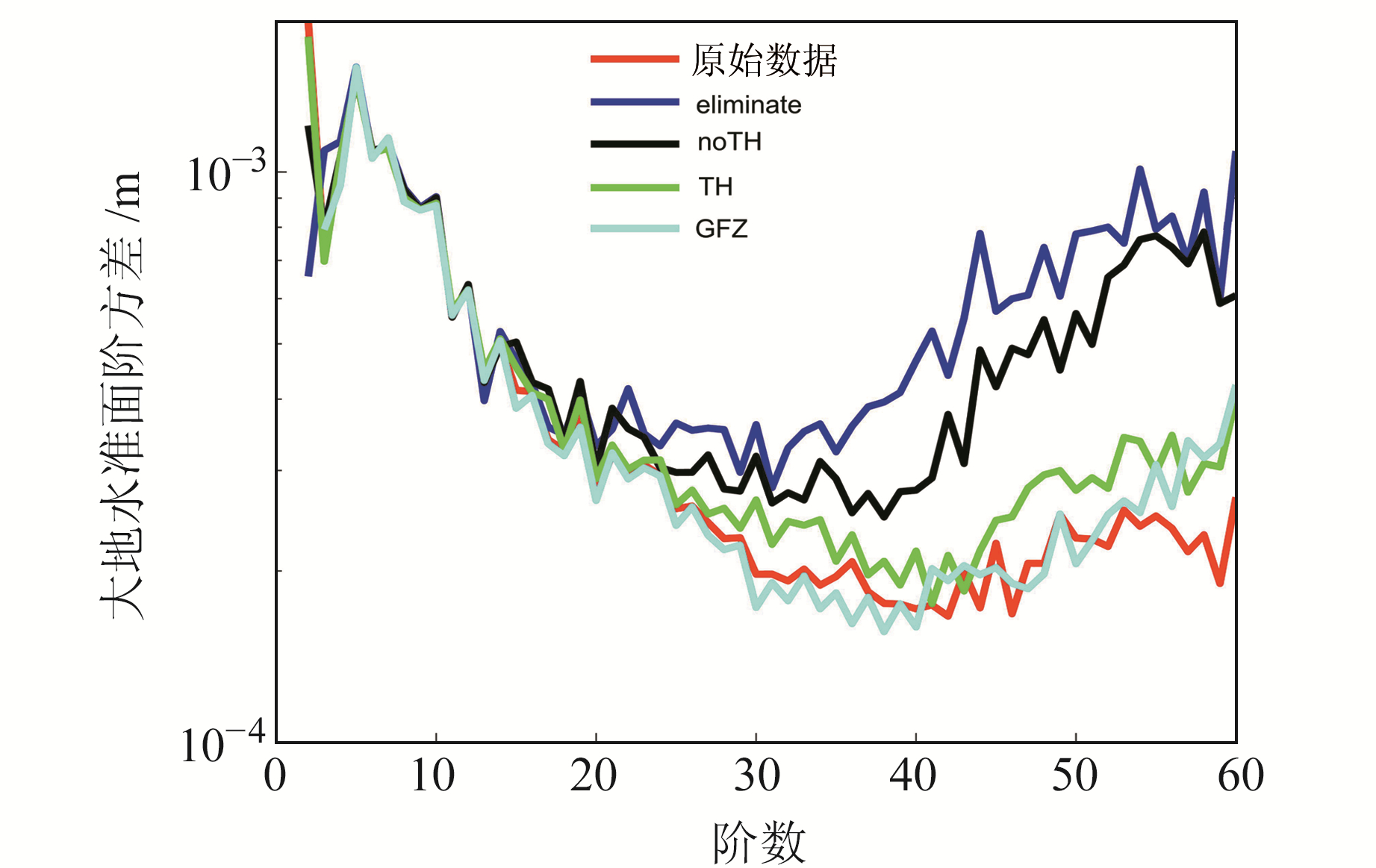﻿ GRACE-FO加速度计校正方法研究文章快速检索 高级检索
 大地测量与地球动力学2021, Vol. 41Issue (10): 998-1003, 1094  DOI: 10.14075/j.jgg.2021.10.002引用本文NIU Hanhan, WANG Changqing, ZHONG Min, et al. Research on Calibration Method of GRACE-FO Accelerometer[J]. Journal of Geodesy and Geodynamics, 2021, 41(10): 998-1003, 1094.Foundation support

National Natural Science Foundation of China, No.41704013; Space Advance Research Strategic Pilot Project of CAS, No.XDA15017700.

第一作者简介

NIU Hanhan, postgraduate, majors in the time-variable gravity field determination, E-mail: niuhan@apm.ac.cn.

文章历史

GRACE-FO加速度计校正方法研究

1. 中国科学院精密测量科学与技术创新研究院大地测量与地球动力学国家重点实验室，武汉市徐东大街340号，430077;
2. 中国科学院大学，北京市玉泉路19号甲，100049;
3. 华中科技大学物理学院，武汉市珞喻路1037号，430074

1 加速度计校正基本理论

1.1 动力学基本方法

 $\mathit{\boldsymbol{\ddot r}}\left( t \right) = \mathit{\boldsymbol{a}}(t;{\mathit{\boldsymbol{r}}_0}, {\mathit{\boldsymbol{\dot r}}_0}, \mathit{\boldsymbol{r}}, \mathit{\boldsymbol{\dot r}}, {\rm{ }}\mathit{\boldsymbol{y}})$ (1)

 $\Delta \mathit{\boldsymbol{r}} = \mathit{\boldsymbol{r}}\left( t \right) - {\mathit{\boldsymbol{r}}_0}\left( t \right) = {\mathit{\boldsymbol{ \boldsymbol{\varPhi} }}_r}\delta ({\mathit{\boldsymbol{r}}_0}, {{\mathit{\boldsymbol{\dot r}}}_0}) + {\mathit{\boldsymbol{S}}_r}\delta \mathit{\boldsymbol{P}}$ (2)

 $\mathit{\boldsymbol{r}}\left( t \right) = {\mathit{\boldsymbol{r}}_0}\left( t \right) + \frac{{\partial {\mathit{\boldsymbol{r}}_0}\left( t \right)}}{{\partial \mathit{\boldsymbol{y}}}}\Delta \mathit{\boldsymbol{y}}$ (3)

 $\mathit{\boldsymbol{\dot \rho }}\left( t \right) = {{\mathit{\boldsymbol{\dot \rho }}}_0}\left( t \right) + \frac{{\partial {{\mathit{\boldsymbol{\dot \rho }}}_0}\left( t \right)}}{{\partial {r_{12}}}}\frac{{\partial {r_{12}}}}{{\partial \mathit{\boldsymbol{x}}}}\Delta \mathit{\boldsymbol{x}}$ (4)

 $\left( {\begin{array}{*{20}{c}} {{\mathit{\boldsymbol{N}}_{{\rm{leo}}}}\mathit{\boldsymbol{y}} = {\mathit{\boldsymbol{b}}_{{\rm{leo}}}}}\\ {{\mathit{\boldsymbol{N}}_{{\rm{kbrr}}}}\mathit{\boldsymbol{y}} = {\mathit{\boldsymbol{b}}_{{\rm{kbrr}}}}} \end{array}} \right)$ (5)

1.2 加速度计偏差参数校正策略

 ${\rm{AC}}{{\rm{C}}_{{\rm{new}}}} = B + S\cdot{\rm{ACC1B}}$ (6)

 $B = {C_0} + {C_1} \times t$ (7)表 1 加速度计偏差参数校正策略 Tab. 1 Accelerometer estimation parameter strategies
1.3 摄动力模型表 2 力模型 Tab. 2 Force models
2 结果分析

2.1 轨道拟合结果表 3 采用不同策略拟合的轨道残差 Tab. 3 Orbit residuals fitted with different strategies图 1 不同策略拟合的轨道3D位置残差 Fig. 1 Orbit 3D position residuals fitted by different strategies

2.2 时变重力场结果 2.2.1 三轴采用同样的校正形式图 2 不同策略反演的重力场 Fig. 2 The gravity field determination by different cases
2.2.2 XYZ轴分别采用不同的校正参数个数表 4 20阶、40阶、60阶重力场累计阶方差(常数偏差参数形式) Tab. 4 Cumulative degree variance of the 20, 40, 60 gravity field(constant strategies)图 3 20、40、60阶重力场累计阶方差(常数偏差形式) Fig. 3 Cumulative degree variance of the 20, 40, 60 gravity field(constant strategies)表 5 20阶、40阶、60阶累计阶方差(线性偏差参数形式) Tab. 5 Cumulative degree variance of the 20, 40, 60 gravity field(linear strategies)图 4 20、40、60阶重力场累计阶方差(线性偏差参数形式) Fig. 4 Cumulative degree variance of the 20, 40, 60 gravity field(linear strategies)图 5 阶方差与模型空间分布 Fig. 5 Degree variance and models spatial distribution
2.3 时变重力场关于加速度计校正的进一步分析与讨论图 6 去除推进器信号后的大地水准面阶方差 Fig. 6 The degree variance after removing the thruster signal
3 结语

  Tapley B D, Bettadpur S, Watkins M, et al. The Gravity Recovery and Climate Experiment: Mission Overview and Early Results[J]. Geophysical Research Letters, 2004, 31(9): L09607 (0)  Christophe B, Boulanger D, Foulon B, et al. A New Generation of Ultra-Sensitive Electrostatic Accelerometers for Follow-on and towards the Next Generation Gravity Missions[J]. Acta Astronautica, 2015, 117: 1-7 DOI:10.1016/j.actaastro.2015.06.021 (0)  Kornfeld R P, Arnold B W, Gross M A, et al. GRACE-FO: The Gravity Recovery and Climate Experiment Follow-on Mission[J]. Journal of Spacecraft and Rockets, 2019, 56(3): 931-951 DOI:10.2514/1.A34326 (0)  王正涛. 卫星跟踪卫星测量确定地球重力场的理论与方法[D]. 武汉: 武汉大学, 2005 (Wang Zhengtao. Theory and Methodology of Earth Gravity Field Recovery by Satellite-to-Satellite Tracking Data[D]. Wuhan: Wuhan University, 2005) (0)  Teixeirada E J, Save H, Tapley B, et al. Accelerometer Parameterization and the Quality of Gravity Recovery and Climate Experiment Solutions[J]. Journal of Spacecraft and Rockets, 2020, 57(4): 740-752 DOI:10.2514/1.A34639 (0)  Klinger B, Mayer-Gürr T. The Role of Accelerometer Data Calibration within GRACE Gravity Field Recovery: Results from ITSG-Grace2016[J]. Advances in Space Research, 2016, 58(9): 1 597-1 609 DOI:10.1016/j.asr.2016.08.007 (0)  王长青. GRACE时变重力场反演与相关问题研究[D]. 武汉: 中国科学院测量与地球物理研究所, 2015 (Wang Changqing. Time-Variable Gravity Field Determination from GRACE Mission and Some Related Issues[D]. Wuhan: Institute of Geodesy and Geophysics, CAS, 2015) (0)  冉将军. 低低跟踪模式重力卫星反演理论、方法及应用[D]. 武汉: 中国科学院测量与地球物理研究所, 2013 (Ran Jiangjun. Theory, Methodology and Application of Recovery Using Low-Low Tracking Gravity Satellite Data[D]. Wuhan: Institute of Geodesy and Geophysics, CAS, 2013) (0)  周浩. 联合多类卫星重力数据反演地球重力场的研究[D]. 武汉: 武汉大学, 2015 (Zhou Hao. Study on the Recovery of the Earth's Gravity Field from the Combination of Multi-Type Satellite Gravimetry Data[D]. Wuhan: Wuhan University, 2015) (0)  Chen Q J, Shen Y Z, Francis O, et al. Tongji-GRACE02s and Tongji-GRACE02k: High-Precision Static GRACE-Only Global Earth's Gravity Field Models Derived by Refined Data Processing Strategies[J]. Journal of Geophysical Research: Solid Earth, 2018, 123(7): 6 111-6 137 DOI:10.1029/2018JB015641 (0)  Bettadpur S. Recommendation for A-Priori Bias and Scale Parameter for Level-1B ACC Data[Z]. 2009 (0)  吴林冲, 衷路萍, 刘冰石. 点火脉冲对GRACE加速度计校准的影响[J]. 大地测量与地球动力学, 2018, 38(4): 399-401 (Wu Linchong, Zhong Luping, Liu Bingshi. Effect of Pulse Thrusting on GRACE Accelerometers Calibration[J]. Journal of Geodesy and Geodynamics, 2018, 38(4): 399-401) (0)  Dahle C, Flechtner F, Murböck M, et al. GRACE-FO D-103919: GFZ Level-2 Processing Standards[S]. GFZ German Research Centre for Geosciences, 2019 (0)
Research on Calibration Method of GRACE-FO Accelerometer
NIU Hanhan1,2WANG Changqing1     ZHONG Min1,2     YAN Yihao1,3     LIANG Lei1
1. State Key Laboratory of Geodesy and Earth's Dynamics, Innovation Academy for Precision Measurement Science and Technology, CAS, 340 Xudong Street, Wuhan 430077, China;
2. University of Chinese Academy of Science, A19 Yuquan Road, Beijing 100049, China;
3. School of Physics, Huazhong University of Science and Technology, 1037 Luoyu Road, Wuhan 430074, China
Abstract: Based on GRACE-FO data of January 2019, using the dynamics method with 24 hours arc length, we analyze results of orbital fitting and time-variable gravity field recovery by adopting different accelerometer parameterization strategies. The results show that: the results of orbital fitting are related to the number of parameters in the accelerometer calibration, and the results would be better with the increasing of accelerometer calibrated parameters. Further, there is not the conspicuous improvement in fitting orbit whatever the strategies are used in directions of the three-axis accelerometer. However, the performance of results of the recovered gravity field is influenced by the number of parameters in the accelerometer calibration, and present some differences if we take different strategies at different directions. Through the cases we investigate, the result would be better if we estimate more accelerometer parameters on the Y direction in SRF frame than other cases, especially, at degrees greater than 40.
Key words: accelerometer; GRACE-FO; dynamic method; bias parameterization